# OPERATORS IN JAVA

Operators In JAVA | Core Java Tutorial | Minigranth

# Operators in Java : Introduction

• Like any other programming language concept of operators in Java exists too. Operators can operate with operands and then produce a result upon operation
• There exists eight different types of operators in java which are discussed listed below and are discussed separately in the later part of this chapter.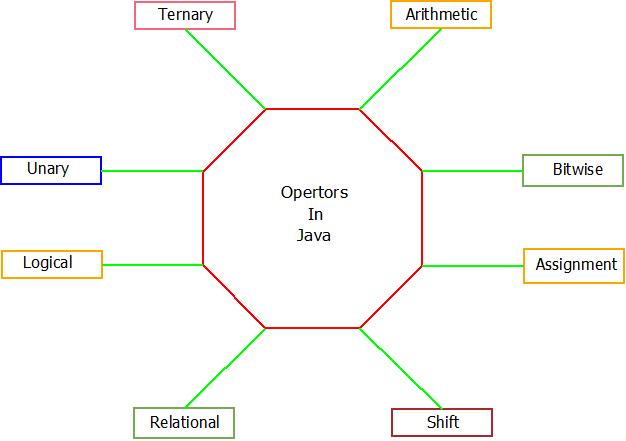Operators In Java : Types

### Operators In Java : Operator Precedence

• The operators in the table are listed below according to the precedence. The one with the highest precedence is on the top and then are of lower precedence and so on.Operator Precedence : Java

• Rules for evaluating a expression containing many operators of same or different precedence are implemented as,
• Operators with higher precedence are operated before.
• In above table operators in same row have same precedence. If in expression the precedence of operators is same then operators are evaluated either left to right or right to left based on their operating direction.
• All binary operators except for the assignment operators are evaluated from left to right; assignment operators are evaluated right to left.
• We have tried to cover all operators with their basic program below in the examples. Refer to below examples and later as you will head in Java you will learn how to use operators.

### Operators In Java : The Discussion

• Below are the examples(programs) for the various operators in java and how to use them.
1. Arithmetic Operators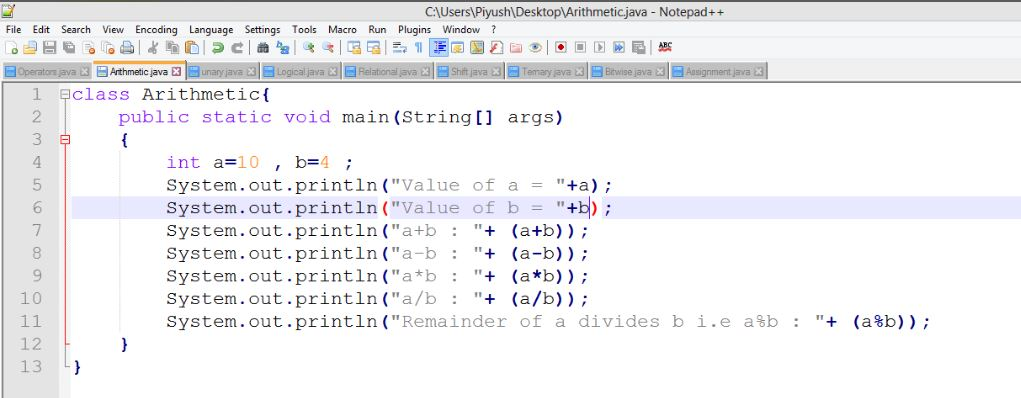Arithmetic Operators : Example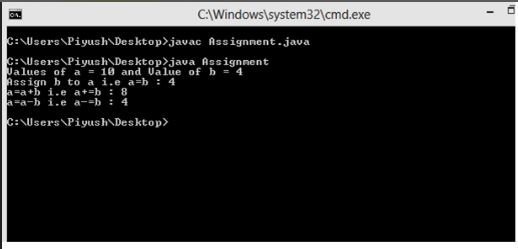Arithmetic Operators : Output

2. Unary OperatorsUnary Operators : Example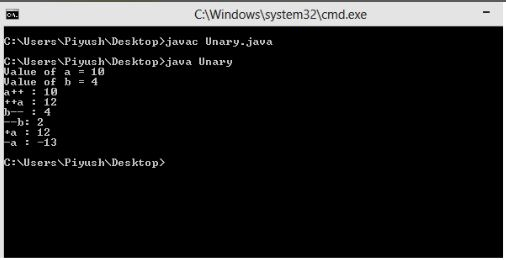Unary Operators : Output

3. Relational Operators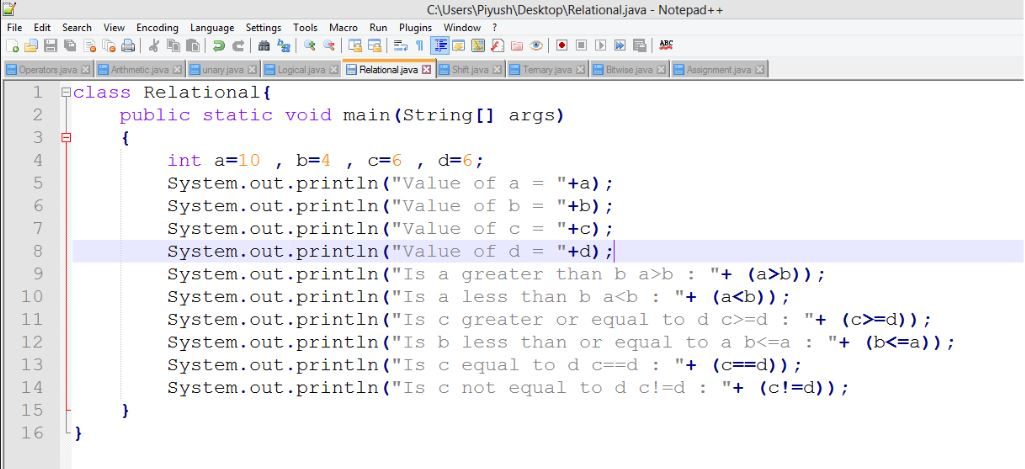Relational Operators : Example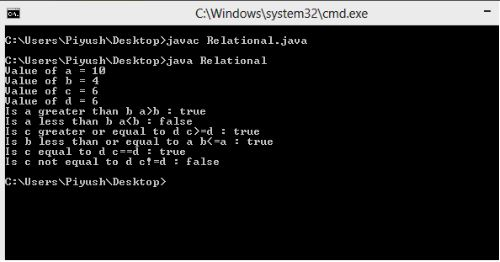Relational Operators : Output

4. Logical OperatorsLogical Operators : ExampleLogical Operators : Output

5. Assignment Operators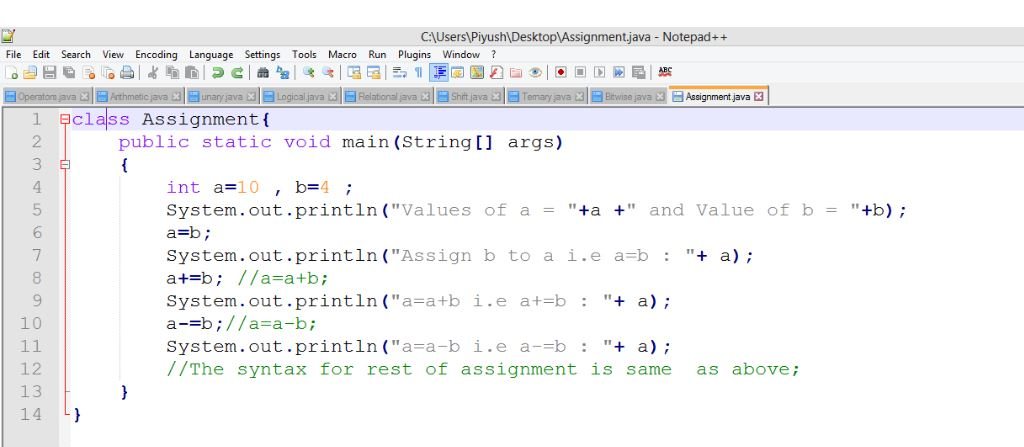Assignment Operators : ExampleAssignment Operators : Example

6. Shift OperatorsShift Operators : Example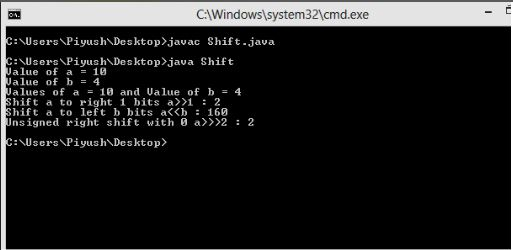Shift Operators : Output

7. Bitwise OperatorsBitwise Operators : ExampleBitwise Operators : Output

8. Ternary OperatorTernary Operators : Example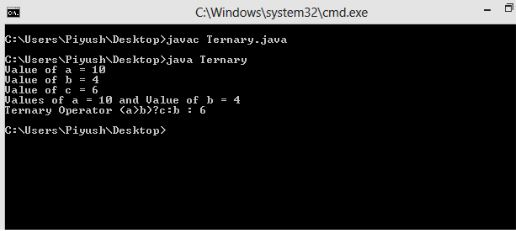Ternary Operators : Output